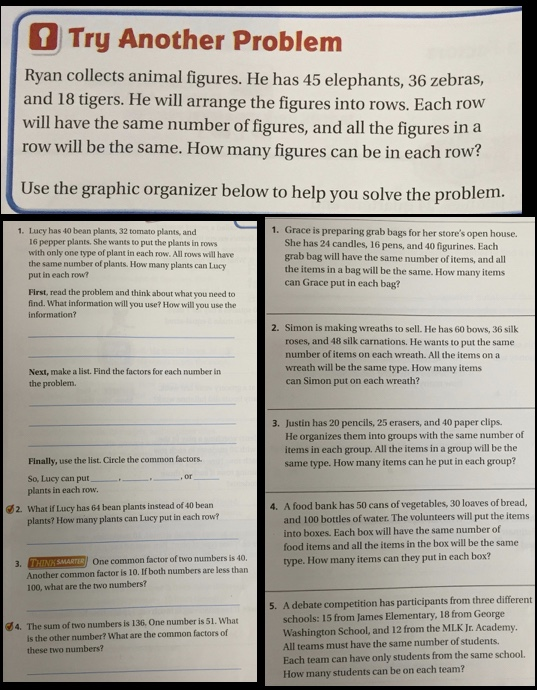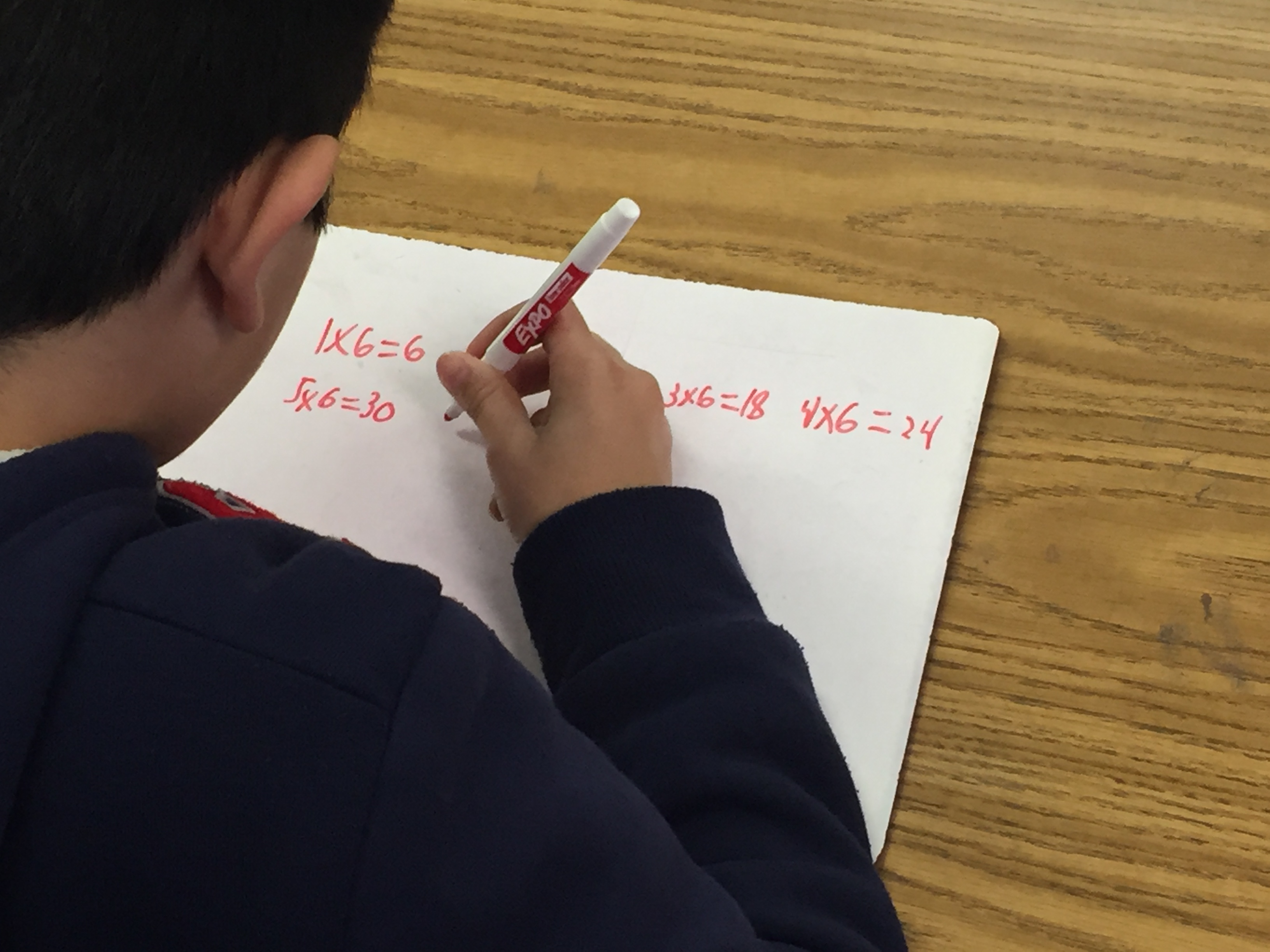# Finding Common Factors: Making Over A Textbook Problem

I had the opportunity to work with a team of three 4th grade teachers the other week.  We were exploring a lesson about finding factors for numbers less than 100 (4.OA.4).  I’d love to hear your input and comments and ways to make this better.  I’m always eager to learn and grow.  My hope is that you also find this post useful in your own work.

We spent 4 hours on Tuesday discussing student engagement and examining how their current curriculum frames the learning experience for students around factors.  (Spoiler alert:  the curriculum sucks life and wonder out of learning.)  It was immediately apparent that the structure of the lesson in the curriculum was incongruent with our vision of a classroom of students engaged in mathematics and producing knowledge (and allowing us to understand and identify their own misconceptions).  So we gave the lesson a makeover and then spent 4 hours on Thursday each teaching the lesson to different groups of 4th graders, observing student learning, and reflecting on our work.

There are a lot of layers to our learning but I want to share (and get feedback) about making over the textbook problem.  If you’re reading this, you are probably already aware of the significant body of work around finding ways to restructure the lessons found in traditional math curriculum and “opening them up” so that students can explore, inquire, and make meaning for themselves.  (Thank you Dan Meyer, Robert Kaplinsky, and Nanette Johnson among many many others!)

The textbook presents the following “essential question” to students:  How can you use the “make a list” strategy to solve problems with common factors?  Ironically (tragically?) the publisher claims that this lesson addresses Math Practice 5:  Use appropriate tools strategically.  That practice asks that “mathematically proficient students consider the available tools when solving a mathematical problem.”  In the EQ, that tool is given; there is nothing for the students to consider.  Can student use tools strategically if we’re telling them what tool to use?

The text then offers this problem as an entry point into common factors:Exciting?  Compelling?  Engaging?

We didn’t think so either.  We discussed many of the obstacles to student learning that were inherent in this problem.

Second, this problem is, for a lack of a better word, lame.  We discussed giving coin manipulative to groups of students so they could create the problem and act it out for themselves.  But we were struck by how arbitrary the problem was.  What’s the point?  Why does Chuck care to do this?  Why would a student want to help Chuck out?

Lastly, the graphic organizer was dauntingly tedious.  The curriculum says that this lesson was also about Math Practice 1.  Presumably the graphic organizer was to teach students how to make sense of the problem and demonstrate perseverance.  We looked at it and asked ourselves, “How are we going to get students to make meaning of this without being a ‘sage on a stage’ leading our students through it?”

Here’s the graphic organizer:There were a lot of things we didn’t like about the organizer.  We felt like it was a really long journey to find common factors and there wasn’t much room for student exploration and discussion.  And the structure of the answer states that there are exactly four answers.  And at the end of the problem, we are still left with a stale “So what?” taste in our mouths.  “We did all that work, for this answer?”  Furthermore, this problem has a closed beginning (every student solves the same problem), a closed middle (every student uses the same organizer and fills in the same blanks), and a closed middle (every student should have the same answers).

The lesson continued in the text with the same similar structure of problems.It’s important to note here that students are not asked to group different things into equal sized groups that have the same ratios of unlike things.  We wanted to believe that’s what was being asked because it’s useful to do such things.  For example, if a food bank asked students to make food boxes containing equal amounts of water, bread, and cans, that’s a worthwhile problem.  But #4 on the right above doesn’t!  It’s asking students to make equal sized boxes where each box contains only water, bread, OR cans.  Why would we want to do that?  And why would we want to spend an hour of math time figuring out problems like this?

So, we refocused our work on the following goals.  We wanted students to solidify their understanding of factors (and how they are related to products).  We wanted students to discover and identify common factors of numbers.  We wanted to see students interacting and cooperating with each other as they do the math and the thinking.  And we wanted to make sure that students of all math levels could participate, learn, and grow.  Low floor + high ceiling = full student engagement.

We created the following pathway.  Each of the three teachers made modifications to this lesson to suit their own student needs.  (If you want to see the lesson plan:  Lesson Inquiry for 4th Grade Factors.)

We started the lesson by asking students:  “Write as many numbers as you can that have 6 as a factor.”

We gave them about 2-3 minutes to do this.  We like the question because it was open.  There are an infinite number of numbers with a factor of 6, albeit only 16 less than a 100.  It was both accessible and challenging because the measure of success was “as many as you can”.

Two amazing things happened.

First, some students wrote something like this:We anticipated this mistake and celebrated it because it answers a related question.  We borrowed Dan Meyer’s question “What other question does this solution answer?”  This allowed the class to celebrate the incorrect answer by showing us what it does teach us while also reviewing factors and products.  It’s also a reminder that constantly asking students the same question in the same format (“List the factors of …”) conditions students to follow a structure at the expense of thinking flexibly.

Second, some kids started writing stuff like this:We saw students developing different strategies for finding numbers that have 6 as a factor.  The students doing similar work above started to make connections between factors and multiples.  Other students started doing skip counting.  Other students dug out their times tables.  Students were able to share strategies and reasoning.

One student suggested that 32 had 6 as a factor.  The discussion around this error was rewarding and enriching.

The teacher recorded student responses on the board and then chose 4 numbers to work with (such as 12, 36, 42, and 96).

Then we asked students to choose a number and list all the factors.  We allowed students to choose the number that challenged them but they also felt like they could struggle productively.  In one class, we had something like this on the board:

12: 1, 2, 3, 4, 6, 12;

36: 1, 2, 3, 4, 6, 9, 12, 18, 36;

42: 1, 2, 3, 6, 7, 14, 21, 42

96: 1, 2, 3, 4, 6, 8, 12, 16, 24, 32, 48, 96

In small groups, students were asked to make statements about what they noticed.  We recorded all the responses on the board and unpacked what we could in the time remaining.  Students identified that they not only all had 6 as a common factor, but also 3 and 2.  We asked why that was and students had a rich discussion.  One student asked:  “If two numbers have 6 as a factor, will they always have 2 and 3 as factors?”  Sweet.  We asked students to make other statements that they thought were true and recorded them for use in the next class.

Below is a thing outline/pathway that we created and use to guide instruction.  Every lesson looked differently in reality, but had similar threads that I’ve described above.

Lesson Inquiry for 4th Grade Factors

Where did we miss?  How could we have done it better?  What might be useful to you?

Interested in your thoughts.  More to come soon.

This site uses Akismet to reduce spam. Learn how your comment data is processed.